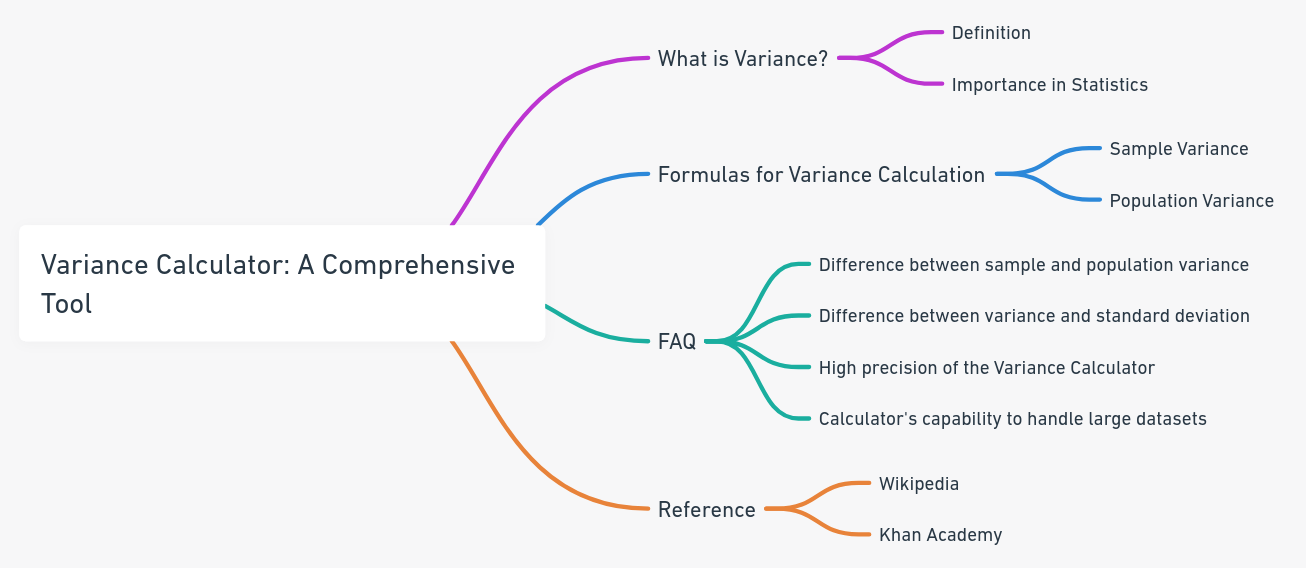Even The mini Tools Can Empower People to Do Great Things.

# Variance Calculator: A Comprehensive Tool for Sample and Population Variance

Discover the high-precision Variance Calculator to compute both sample and population variance. Step-by-step instructions and formulas included.

Variance Calculator (High Precision)

Enter numbers separated by comma [example1], space [example2] or line break [example3]:

If your text has non-numeric content, use our Number Extractor for cleaner calculations.
 Precision: 10 20 30 40 50 60 70 80 90 100 200 300 400 500 600 700 800 900 1000

Embed Variance Calculator (High Precision) Widget

## About Variance Calculator (High Precision)The Variance Calculator is a tool designed to compute the variance of a dataset quickly and accurately. Whether you're working with a sample or an entire population, understanding the variance can help in various fields, from finance to science. Our Variance Calculator stands out due to its step-by-step and high-precision features, ensuring you have the insights you need.

## What is Variance?

Variance is a statistical measure that represents the dispersion or spread of a set of data points. In simpler terms, it tells us how much each number in a dataset varies from the mean (average) of that dataset. Variance is crucial in statistics as it provides insights into the data's variability, helping researchers and analysts make informed decisions.

## Formulas for Variance Calculation:

### 1. Sample Variance:

$s^2 = \frac{\sum (x_i - x̄)^2}{N-1}$

Where:

1. $$s^2$$ = sample variance
2. $$x_i$$ = individual data points in the sample
3. $$x̄$$ = mean value of the sample data set
4. $$N$$ = size of the sample data set

### 2. Population Variance:

$σ^2 = \frac{\sum (x_i - μ)^2}{N}$

Where:

1. $$σ^2$$ = population variance
2. $$x_i$$ = individual data points in the population
3. $$μ$$ = mean of the population data set
4. $$N$$ = size of the population data set

Understanding variance and using the right formula is essential for accurate data analysis. Whether you're a student, researcher, or professional, the Variance Calculator can be a valuable tool in your statistical toolkit.

## FAQ

Sample variance is derived from a subset of the population, while population variance considers the entire population. The formulas for both are slightly different.

Yes, while both measure the spread of data, variance is the average of squared deviations from the mean, and standard deviation is the square root of variance.

The Variance Calculator is meticulously engineered to deliver unparalleled accuracy, boasting the capability to calculate results up to 1000 decimals. This level of precision ensures that users receive the most exact variance values for their dataset, making it an indispensable tool for those who require detailed and highly accurate statistical computations.

Our Variance Calculator is not only precise but also robust. It's designed to handle extensive datasets with ease. In our rigorous testing, we input a set of 50,000 numbers, and the Variance Calculator delivered the results instantly. This showcases its capability to manage large number sets efficiently, making it a top choice for professionals and researchers dealing with comprehensive datasets.

## Reference

Reference this content, page, or tool as:

"Variance Calculator (High Precision)" at https://miniwebtool.com/variance-calculator/ from miniwebtool, https://miniwebtool.com/

by miniwebtool team. Updated: Oct 07, 2023